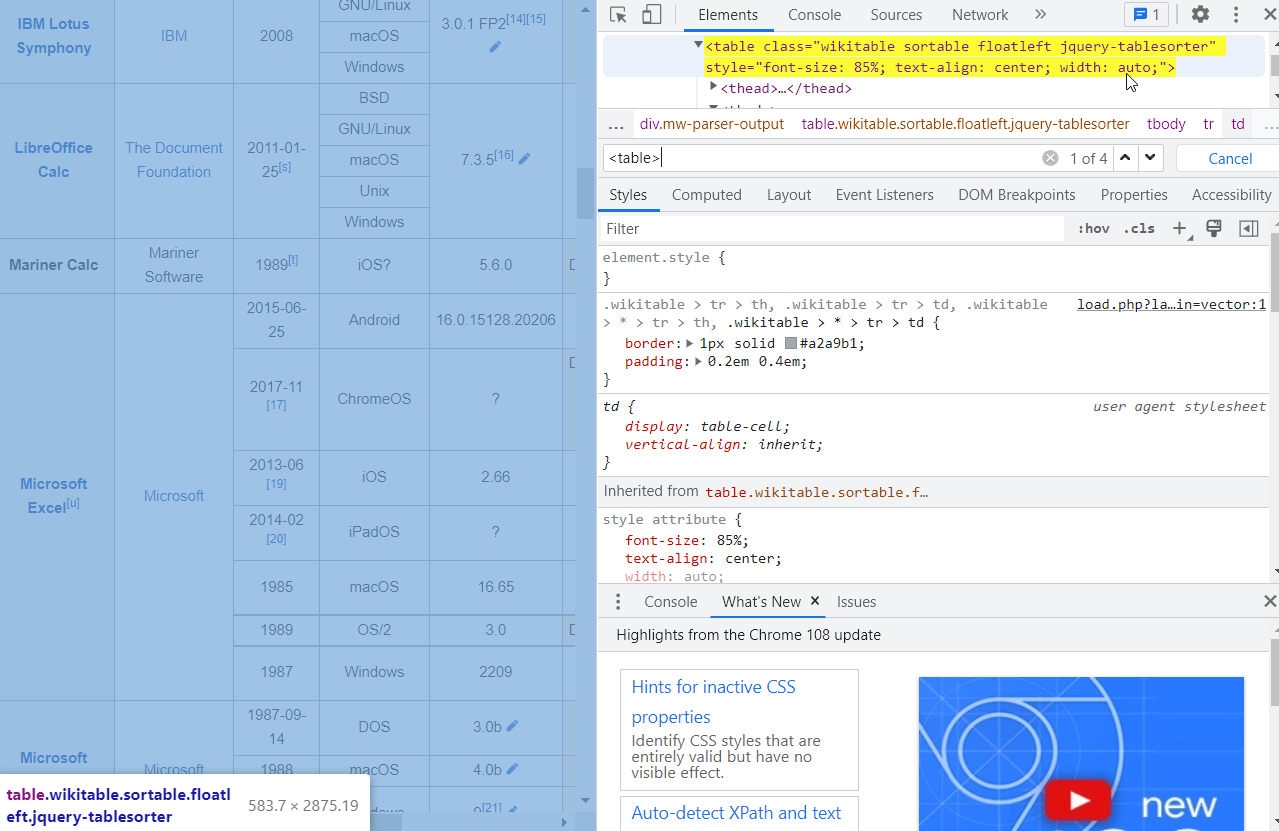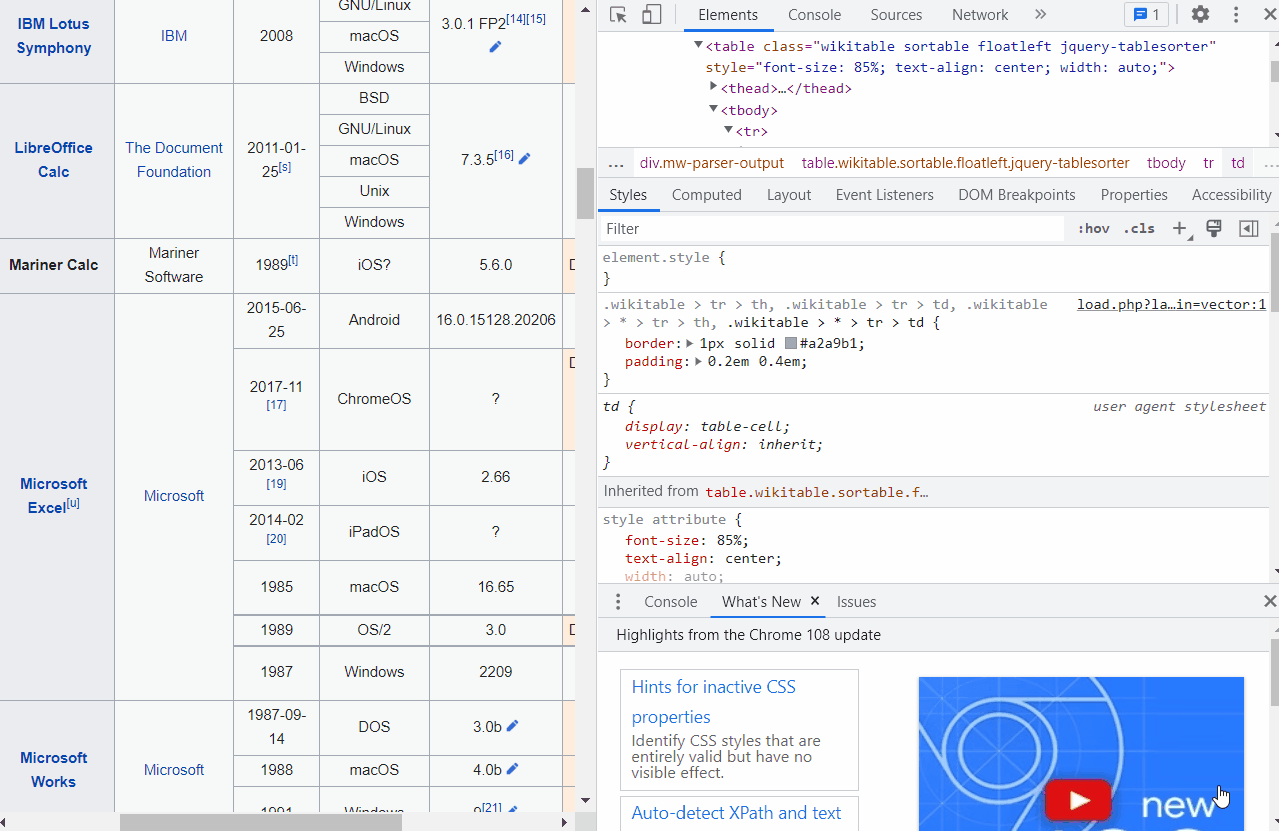# IMPORTHTML Google Sheets - The Ultimate Guide for 2023

10 MinutesIn this article we will show you exactly how to use the IMPORTHTML function in Google Sheets. The IMPORTHTML function is used to import tables and lists from webpages to Google Sheets. You will learn step-by-step how to identify the index of the table or list to import, and then use this function to import them to Google Sheets.

### How to Import Whole Table or List

=IMPORTHTML(url, query, index)

Syntax Breakdown:

=IMPORTHTML: The function used for importing html tables or lists.

Url: The URL to the web page, enclosed in double quotes

Query: The type of data you want to import. There are two choices: table and list. Enclose it in double quotes.

Index: Web pages can contain multiple tables and/or lists. The index helps Google Sheets identify which instance to import.

### Import Only Portion of Table

=QUERY(IMPORTHTML(url, query, index), command)

Syntax Breakdown:

=IMPORTHTML: the function used for importing the table from a webpage

Url: the URL to the web page

Query: the type of data you want to import. There are two choices: table and list.

Index: Web pages can contain multiple tables and/or lists. The index helps Google Sheets identify which instance to import.

=QUERY: the function used for filtering the data to import

Command: the specific QUERY command to set the portion of the table to import. You can limit the rows or columns to import using this command.

We will have two examples using this function combined with QUERY. You can try more QUERY commands by learning about its basics here

## How to Identify the Index of the Table or List

Web pages often contain tables and lists that are displayed differently, and they can be imported using the IMPORTHTML function. To ensure that the right table or list is selected, you should be able to identify its corresponding index.

The index of a table or list identifies which of the tables or lists in the webpage will be imported. For example, setting the index equal to 1 means you want to import the first table or list in the webpage. Setting the index to be equal to 2 means you want to import the second table or list in the webpage, and so on.

Below are the steps to do so, using the Chrome browser. Most browsers operate similarly so you should be able to easily do so using other browsers.

#### 2. Select Inspect

The Inspect panel will appear on the right side of the browser.

#### 3. Press Ctrl+F Keyboard Shortcut

Click the scroll bar first on the upper-right panel of the screen so that the search bar will appear on the right place once you press Ctrl+F.

#### 4. Type “<table>” for tables and “<ul>” for listsIdentify table index, source webpage inspect element, search for table tag

#### 5. Click the Next Button Until the Right Table or List is Highlighted

Once the table or list you want to import is highlighted, write down its corresponding number and that is its index. For our example here, the number is 1 of 4. The number 1 is the index of the table.

Web pages may contain hidden tables and lists for formatting the page and they can still be imported by the function. Thus it may not be possible to simply visually just count the number of tables or lists and then identify the order the target table or list appears. This is the best method to make sure you identify the correct index.Identify table index, source webpage inspect element, search for the table index screencap

### Import a table from a web page

#### 1. Identify URL query, and index

Query: table

Index: 1

Use the steps in the previous section “Identify the Index of a Table” to determine how to find the index of a table.

#### 2. Select a cell and add formula =IMPORTHTML(url, query, index)

We will add the formula in the first cell of the sheet A1. The formula is

### Import Only the First Few Rows of a Table

In this example, we want to import the list of web-based spreadsheets from the Wikipedia page on spreadsheets (https://en.wikipedia.org/wiki/Comparison_of_spreadsheet_software). For this case, we will only import the first five rows plus the header row. Here are the steps to do so:

#### 1. Identify URL, query, and index

Query: table

Index: 1

Command

Use the steps in the previous section “Identify the Index of a Table” to determine how to find the index of a table.

#### 2. Use the QUERY command to limit rows

Next we will use the QUERY command to limit the number of rows we will import by using:

limit N

To count the actual number of rows to import, it is best to check the web page itself first. It is common for rows to have subdivisions within them. These are actually small rows in one column being merged in other columns. You should be sure to count the smallest divisions.

For our example, we want to import the first 5 entries which, on our first thought, will be 5 rows. But if we look at the 4th and 5th rows there are multiple rows within them. As the 4th column has the biggest number of divisions, we will count them one-by-one. The number of rows is 24.

The final command is

Limit 24

#### 3. Select the cell and add formula =QUERY(IMPORTHTML(url, query, index), command)

We will add the formula in the first cell of the sheet A1. The formula is

### Import Only Selected Columns of a Table

In this example, we want to import the list of web-based spreadsheets from the Wikipedia page on spreadsheets (https://en.wikipedia.org/wiki/Comparison_of_spreadsheet_software). This time, however, we want to just import the first two columns of the table. Here are the steps to do so:

#### 1. Identify URL Query, and Index

Query: table

Index: 1

Command

Use the steps in the previous section “Identify the Index of a Table” to determine how to find the index of a table.

#### 2. Set up the QUERY command to select required columns

Next, set up the QUERY command. To select the columns use

Select Col1, Col2, ColN

The select command is used to limit the columns to import. This time we only want to import the first two columns so the command becomes:

Select Col1, Col2

Select the cell and add formula =QUERY(IMPORTHTML(url, query, index), command)

We will add the formula in the first cell of the sheet A1. The formula is

### Import a list from a web page

#### 1. Identify URL query, and index

Query: list

Index: 8

Use the steps in previous section “Identify the Index of a List” to determine how to find the index of a list.

#### 2. Select a cell and add formula =IMPORTHTML(url, query, index)

We will add the formula in the first cell of the sheet A1. The formula is

## IMPORTHTML Google Sheets Common Errors

### Google Sheets IMPORTHTML Not Working

There are several reasons IMPORTHTML formula is not working:

• URL changed - check if the URL is still up to date
• Incorrect index - check the web page for changes in the content
• Website is configured to block bots - other methods should be used to access the data

This error occurs because several webpages, especially those that regularly update their content, are generated by scripts that assemble data from different sources to a single page. The scripts can take time to run and the IMPORTHTML formula cannot work.

If what you need is to import analytics data from your e-Commerce and marketing platforms to Google Sheets, this function is not the solution. We recommend Lido. There’s no need to worry about whether the table or list you need will be imported. Instead, you just need to do a few clicks and the data will seamlessly load in your spreadsheet.

Google Sheets automatically updates IMPORTHTML formula every 1 hour. You can adjust the refresh rate to once every 5 minutes. Here are the steps:

#### 1. Add formula =IMPORTHTML(“url?refresh=” & cell, query, index)

Instead of simply using the set formula, you need to add the “?refresh=” portion to it. For this example, we will import the table of Currencies from Yahoo Finance page. The following are the information we need to form the formula:

Url: https://finance.yahoo.com/currencies

Cell: A1

Query: table

Index: 1

The formula becomes

=importhtml("https://finance.yahoo.com/currencies?refresh=" & A1,"table",1)

And we will place the formula in B1.

#### 3. Open Google Sheets Apps Script

To access Google Sheets Apps Script, go to Extensions and select Apps Script

#### 4. Paste the custom script to Apps Script

Paste the following script to Apps Script:

function myFunction() {

var cell = sheet.getRange("A1");

var refresh = parseInt(cell.getValue().toString());

var increment = refresh + 1;

cell.setValue(increment);

}

#### 5. Add trigger to the function

Click Triggers on the left panel.

Afterwards, click the Add trigger button on the lower-right corner of the screen.

A list of options will appear. Use the following settings:

Choose which function to run: myFunction

Choose which deployment should run: Head

Select event source: Time-driven

Select type of time-based trigger: Minutes timer

Select minute interval: Every 5 minutes

Failure notification settings: Notify me daily

Then click Save.

#### 6. Authorize the script

A new window will pop up for authorizing the script to access your sheet. Choose your Google account:

Click Advanced then click Go to Untitled Project (unsafe).

Approve the necessary permissions by clicking:

#### 7. Run the code

Go back to the script by clicking Editor on the left panel. Click Run.